Home > A2C > Chapter 11 > Lesson 11.2.4 > Problem11-92

11-92.
1. For the polynomial function f(x) = x3 − 5x2 + 11x − 15, which of the following are possible factors? Homework Help ✎

1. (x + 1)

2. (x − 2)

3. (x + 4)

4. (x − 3)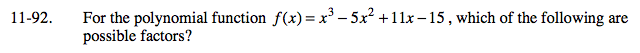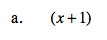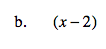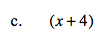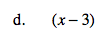Which of the constants in these polynomials are factors of 15.

(a), (d)